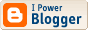## John Baez on Lambda Calculus and Games

John Baez has an intriguing introduction to lambda calculus and games. He converts a game tree into an expression built from products, exponents, and a single term X (hence there is a close connection to CCCs). The corresponding game is unwinnable if setting X=0 and evaluating yields 0 (so X is an unwinnable game: there are no values of type All X.X) and winnable if evaluating yeilds 1 (so X^X is a winnable game: there is a value of type All X. X -> X). The note is a couple of pages long, and ends with a fine survey of introductory material on lambda calculus.